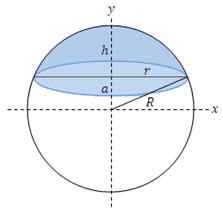## How to Calculate and Solve for the Radius, Height and Surface Area of a Spherical Segment | The Calculator EncyclopediaThe image above is a spherical segment.

To compute the surface area of a spherical segment requires two essential parameters which are the radius of the sphere (R) and the height (h).

The formula for calculating the surface area of the spherical segment:

A = 2πRh

Where;
A = Surface area of the spherical segment
R = Radius of the sphere
h = Height of the spherical segment

Let’s solve an example;
Find the surface area of a spherical segment when the radius of the sphere is 12 cm and the height is 16 cm.

This implies that;
R = Radius of the sphere = 12 cm
h = Height of the spherical segment = 16 cm

A = 2πRh
A = 2π (12 x 16)
A = 2π (192)
A = 6.28 (192)
A = 1206.37

Therefore, the surface area of the spherical segment is 1206.37 cm2.

Calculating the Radius of the Sphere using the Surface Area of the Spherical Segment and the Height.

R = A / 2πh

Where;
R = Radius of the sphere
A = Surface area of the spherical segment
h = Height of the spherical segment

Let’s solve an example;
Find the radius of a sphere with a surface area of 300 cm2 and a height of 12 cm.

This implies that;
A = Surface area of the spherical segment = 300 cm2
h = Height of the spherical segment = 12 cm

R = A / 2πh
R = 300 / 2 x π x 12
R = 300 / 75.41
R = 3.978

Therefore, the radius of the sphere is 3.978 cm.